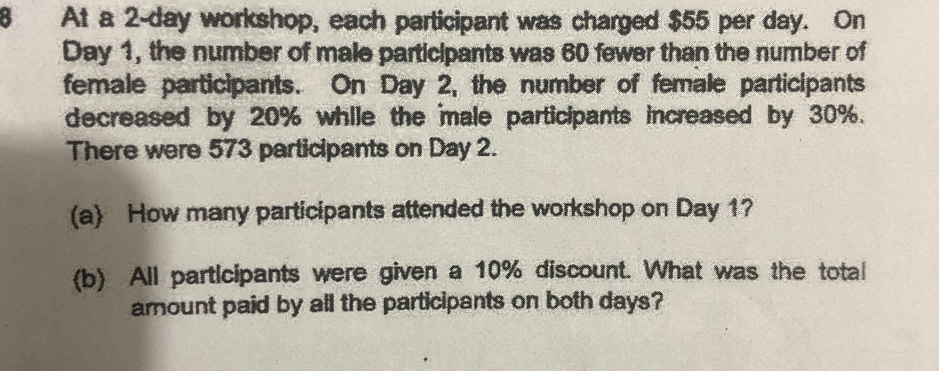# QuestionSource: Nanyang Primary

This is a Matrix question.

 Day 1 Day 2 Total Male 100u 130u Female 100u + 60 80u + 48 200u + 60 210u + 48

210u + 48 = 573

210u = 573 – 48 = 525

1u = 525 ÷ 210 = 2.5

a)

200u = 200 × 2.5 = 500

500 + 60 = 560

b)

560 +573 = 1138

55 × 90% = 49.50

1138 × 49.5 = \$56331

0 Replies 0 Likes